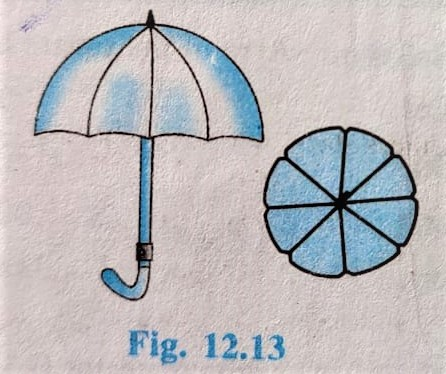# Class 10th Circles## NCERT Area Related to Circle Class 10 Chapter 12 Exercise – 12.2 Solution

Exercise – 12.2 Q1. Find the area of a sector of a circle with radius 6 cm  if angle of the sector is 60º. Solution: Radius (r) = 6 cm θ = 60° Area of Minor Sector = (θ /360) × π r² ⇒  Area of Minor Sector = (60/360) × 22/7 × (6)² ⇒ Area …